Win up to 100% scholarship on Aakash BYJU'S JEE/NEET courses with ABNAT Win up to 100% scholarship on Aakash BYJU'S JEE/NEET courses with ABNAT

# Semiconductors

## What Are Semiconductors?

Semiconductors are materials which have a conductivity between conductors (generally metals) and non-conductors or insulators (such as ceramics). Semiconductors can be compounds, such as gallium arsenide, or pure elements, such as germanium or silicon. Physics explains the theories, properties and mathematical approach related to semiconductors.

Examples of Semiconductors

Gallium arsenide, germanium and silicon are some of the most commonly used semiconductors. Silicon is used in electronic circuit fabrication, and gallium arsenide is used in solar cells, laser diodes, etc.

## Holes and Electrons in Semiconductors

Holes and electrons are the types of charge carriers accountable for the flow of current in semiconductors. Holes (valence electrons) are the positively charged electric charge carrier, whereas electrons are the negatively charged particles. Both electrons and holes are equal in magnitude but opposite in polarity.

### Mobility of Electrons and Holes

In a semiconductor, the mobility of electrons is higher than that of the holes. It is mainly because of their different band structures and scattering mechanisms.

Electrons travel in the conduction band, whereas holes travel in the valence band. When an electric field is applied, holes cannot move as freely as electrons due to their restricted movement. The elevation of electrons from their inner shells to higher shells results in the creation of holes in semiconductors. Since the holes experience stronger atomic force by the nucleus than electrons, holes have lower mobility.

The mobility of a particle in a semiconductor is more, if

• The effective mass of particles is lesser
• The time between scattering events is more

For intrinsic silicon at 300 K, the mobility of electrons is 1500 cm2 (V∙s)-1, and the mobility of holes is 475 cm2 (V∙s)-1.

The bond model of electrons in silicon of valency 4 is shown below. Here, when one of the free electrons (blue dots) leaves the lattice position, it creates a hole (grey dots). This hole thus created takes the opposite charge of the electron and can be imagined as positive charge carriers moving in the lattice.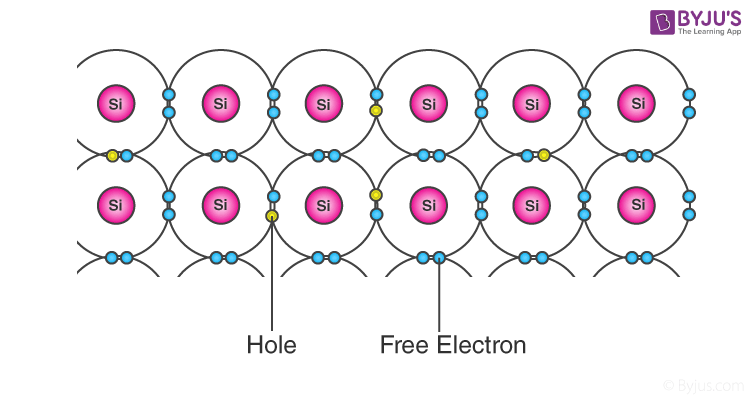Concept of Electrons and Holes in Semiconductors

## Band Theory of Semiconductors

The introduction of band theory happened during the quantum revolution in science. Walter Heitler and Fritz London discovered the energy bands.

We know that the electrons in an atom are present at different energy levels. When we try to assemble a lattice of a solid with N atoms, each level of an atom must split into N levels in the solid. This splitting of sharp and tightly packed energy levels forms Energy Bands. The gap between adjacent bands representing a range of energies that possess no electron is called a Band Gap.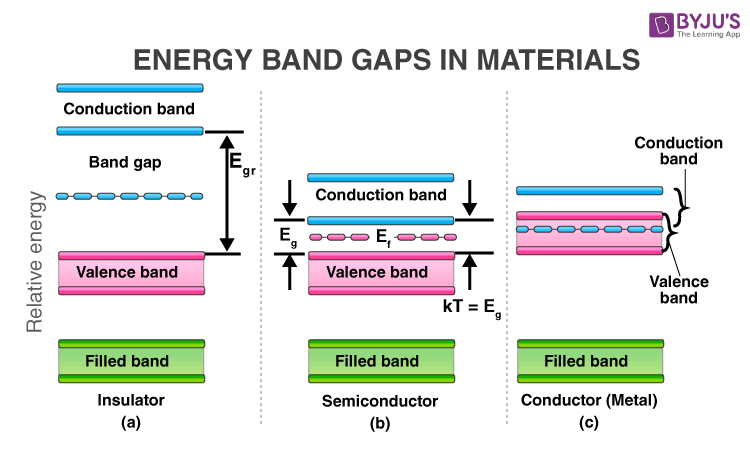Energy Band Diagram for Semiconductors, Conductors and Insulators

### Conduction Band and Valence Band in Semiconductors

#### Valence Band

The energy band involving the energy levels of valence electrons is known as the valence band. It is the highest occupied energy band. When compared with insulators, the band gap in semiconductors is smaller. It allows the electrons in the valence band to jump into the conduction band on receiving any external energy.

#### Conduction Band

It is the lowest, unoccupied band that includes the energy levels of positive (holes) or negative (free electrons) charge carriers. It has conducting electrons resulting in the flow of current. The conduction band possess a high energy level and is generally empty. The conduction band in semiconductors accepts the electrons from the valence band.

### What Is the Fermi Level in Semiconductors?

The Fermi level (denoted by EF) is present between the valence and conduction bands. It is the highest occupied molecular orbital at absolute zero. The charge carriers in this state have their own quantum states and generally do not interact with each other. When the temperature rises above absolute zero, these charge carriers will begin to occupy states above the Fermi level.

In a p-type semiconductor, there is an increase in the density of unfilled states. Thus, accommodating more electrons at the lower energy levels. However, in an n-type semiconductor, the density of states increases, therefore, accommodating more electrons at higher energy levels.

## Properties of Semiconductors

Semiconductors can conduct electricity under preferable conditions or circumstances. This unique property makes it an excellent material to conduct electricity in a controlled manner as required.

Unlike conductors, the charge carriers in semiconductors arise only because of external energy (thermal agitation). It causes a certain number of valence electrons to cross the energy gap and jump into the conduction band, leaving an equal amount of unoccupied energy states, i.e., holes. The conduction due to electrons and holes is equally important.

• Resistivity: 10-5 to 106 Ωm
• Conductivity: 105 to 10-6 mho/m
• Temperature coefficient of resistance: Negative
• Current flow: Due to electrons and holes

### Why Does the Resistivity of Semiconductors Go Down with Temperature?

The difference in resistivity between conductors and semiconductors is due to their difference in charge carrier density.

The resistivity of semiconductors decreases with temperature because the number of charge carriers increases rapidly with an increase in temperature, making the fractional change, i.e., the temperature coefficient negative.

### Some Important Properties of Semiconductors

1. Semiconductors act like insulators at zero Kelvin. On increasing the temperature, they work as conductors.
2. Due to their exceptional electrical properties, semiconductors can be modified by doping to make semiconductor devices suitable for energy conversion, switches and amplifiers.
3. Lesser power losses.
4. Semiconductors are smaller in size and possess less weight.
5. Their resistivity is higher than conductors but lesser than insulators.
6. The resistance of semiconductor materials decreases with an increase in temperature and vice-versa.

## Types of Semiconductors

Semiconductors can be classified as follows:

• Intrinsic Semiconductor
• Extrinsic Semiconductor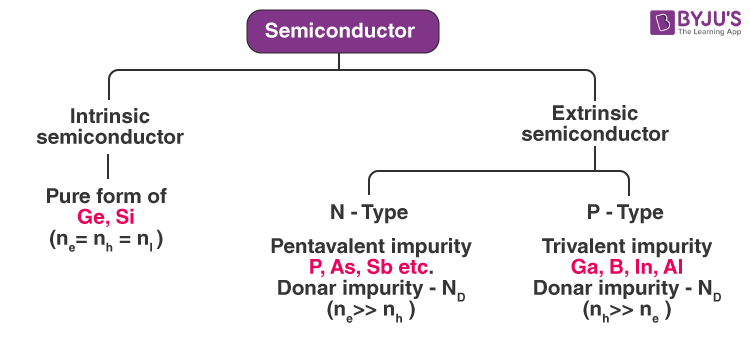Classification of Semiconductors

## Intrinsic Semiconductor

An intrinsic type of semiconductor material is made to be very pure chemically. It is made up of only a single type of element.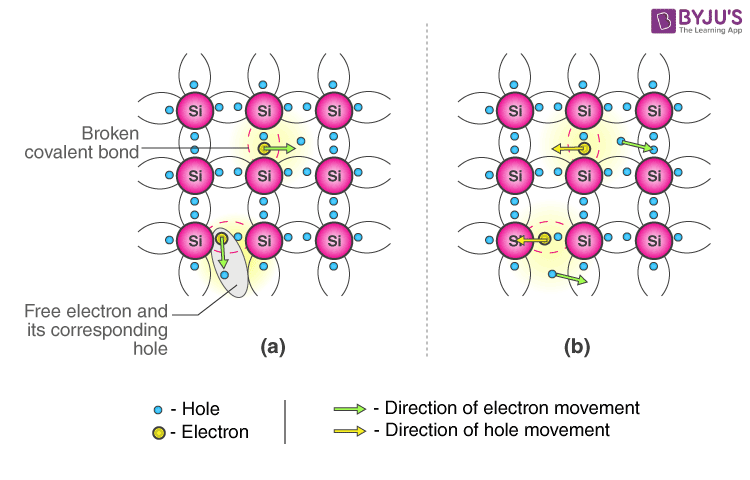Conduction Mechanism in Case of Intrinsic Semiconductors (a) In the absence of an electric field (b) In the presence of an electric field

Germanium (Ge) and silicon (Si) are the most common types of intrinsic semiconductor elements. They have four valence electrons (tetravalent). They are bound to the atom by a covalent bond at absolute zero temperature.

When the temperature rises due to collisions, few electrons are unbounded and become free to move through the lattice, thus creating an absence in its original position (hole). These free electrons and holes contribute to the conduction of electricity in the semiconductor. The negative and positive charge carriers are equal in number.

The thermal energy is capable of ionising a few atoms in the lattice, and hence, their conductivity is less.

### The Lattice of Pure Silicon Semiconductor at Different Temperatures

• At absolute zero Kelvin temperature: At this temperature, the covalent bonds are very strong, there are no free electrons, and the semiconductor behaves as a perfect insulator.
• Above absolute temperature: With an increase in temperature, a few valence electrons jump into the conduction band, and hence, it behaves like a poor conductor.

### Energy Band Diagram of Intrinsic Semiconductor

The energy band diagram of an intrinsic semiconductor is shown below.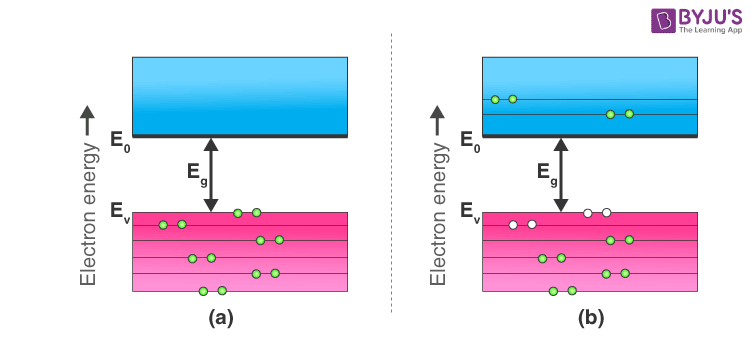(a) Intrinsic Semiconductor at T = 0 Kelvin, behaves like an insulator (b) At t>0, four thermally generated electron pairs

In intrinsic semiconductors, current flows due to the motion of free electrons, as well as holes. The total current is the sum of the electron current Ie due to thermally generated electrons and the hole current Ih.

Total Current (I) = Ie + Ih

For an intrinsic semiconductor, at finite temperature, the probability of electrons existing in a conduction band decreases exponentially with an increasing band gap (Eg).

n = n0e-Eg/2.Kb.T

Where,

## Extrinsic Semiconductor

The conductivity of semiconductors can be greatly improved by introducing a small number of suitable replacement atoms called IMPURITIES. The process of adding impurity atoms to the pure semiconductor is called DOPING. Usually, only 1 atom in 107 is replaced by a dopant atom in the doped semiconductor. An extrinsic semiconductor can be further classified into types:

• N-type Semiconductor
• P-type Semiconductor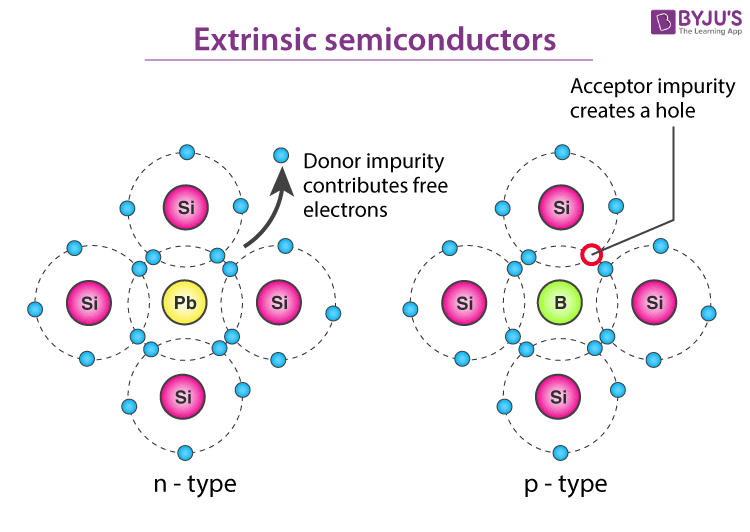Classification of Extrinsic Semiconductor

### N-Type Semiconductor

• Mainly due to electrons
• Entirely neutral
• I = Ih and nh >> ne
• Majority – Electrons and Minority – Holes

When a pure semiconductor (silicon or germanium) is doped by pentavalent impurity (P, As, Sb, Bi), then four electrons out of five valence electrons bond with the four electrons of Ge or Si.

The fifth electron of the dopant is set free. Thus, the impurity atom donates a free electron for conduction in the lattice and is called a “Donar“.

Since the number of free electrons increases with the addition of an impurity, the negative charge carriers increase. Hence, it is called an n-type semiconductor.

Crystal as a whole is neutral, but the donor atom becomes an immobile positive ion. As conduction is due to a large number of free electrons, the electrons in the n-type semiconductor are the MAJORITY CARRIERS, and holes are the MINORITY CARRIERS.

### P-Type Semiconductor

• Mainly due to holes
• Entirely neutral
• I = Ih and nh >> ne
• Majority – Holes and Minority – Electrons

When a pure semiconductor is doped with a trivalent impurity (B, Al, In, Ga), then the three valence electrons of the impurity bond with three of the four valence electrons of the semiconductor.

This leaves an absence of electron (hole) in the impurity. These impurity atoms which are ready to accept bonded electrons are called “Acceptors“.

With an increase in the number of impurities, holes (the positive charge carriers) are increased. Hence, it is called a p-type semiconductor.

Crystal, as a whole, is neutral, but the acceptors become an immobile negative ion. As conduction is due to a large number of holes, the holes in the p-type semiconductor are MAJORITY CARRIERS, and electrons are MINORITY CARRIERS.

## Difference between Intrinsic and Extrinsic Semiconductors

 Intrinsic Semiconductor Extrinsic Semiconductor Pure semiconductor Impure semiconductor The density of electrons is equal to the density of holes The density of electrons is not equal to the density of holes Electrical conductivity is low Electrical conductivity is high Dependence on temperature only Dependence on temperature, as well as on the amount of impurity No impurities Trivalent impurity and pentavalent impurity

## Applications of Semiconductors

Let us now understand the uses of semiconductors in daily life. Semiconductors are used in almost all electronic devices. Without them, our life would be much different.

Their reliability, compactness, low cost and controlled conduction of electricity make them ideal to be used for various purposes in a wide range of components and devices. Transistors, diodes, photosensors, microcontrollers, integrated chips and much more are made up of semiconductors.

### Uses of Semiconductors in Everyday Life

• Temperature sensors are made with semiconductor devices.
• They are used in 3D printing machines
• Used in microchips and self-driving cars
• Used in calculators, solar plates, computers and other electronic devices.
• Transistors and MOSFET used as a switch in electrical circuits are manufactured using semiconductors.

### Industrial Uses of Semiconductors

The physical and chemical properties of semiconductors make them capable of designing technological wonders like microchips, transistors, LEDs, solar cells, etc.

The microprocessor used for controlling the operation of space vehicles, trains, robots, etc., is made up of transistors and other controlling devices, which are manufactured by semiconductor materials.

## Importance of Semiconductors

Here, we have discussed some advantages of semiconductors, which make them highly useful everywhere.

• They are highly portable due to their small size
• They require less input power
• Semiconductor devices are shockproof
• They have a longer lifespan
• They are noise-free while operating

## Practice Problems

1. The energy of a photon of sodium light (λ = 589 nm) equals the band gap of semiconducting material. Find:

• The minimum energy E required to create a hole-electron pair. (5890 A0)
• The value of E/kT at a temperature of 300 K. (81)

2. A P-type semiconductor has an acceptor level of 57 meV above the valence band. What is the maximum wavelength of light required to create a hole? (217100 A0)

## Semiconductors Video Lesson – Important Topics## Semiconductors Important JEE Main Questions### Semiconductor Electronics – Top 12 Most Expected JEE Questions## Frequently Asked Questions on Semiconductors

Q1

### Pure silicon semiconductor at 500K has equal electrons and holes (1.5 × 1016 m-3). Doping by indium increases nh to 4.5 × 1022 m-3. Calculate the type and electron concentration of the doped semiconductor.

Since, n2i = ne nh

(1.5 × 1016)2 = ne (4.5 × 1022)

Therefore, ne = 5 × 109

Given nh = 4.5×1023

⇒ nh >> ne

Therefore, the semiconductor is p-type and ne = 5 × 109 m-3.

Q2

### Why is the valence band in semiconductors partially empty, and the conduction band is partially filled at room temperature?

In semiconductors, the conduction band is empty, and the valence band is completely filled at zero Kelvin. No electron from the valence band can cross over to the conduction band at this temperature. But at room temperature, some electrons in the valence band jump over to the conduction band due to a small forbidden gap, i.e., 1 eV.

Q3

### In an intrinsic semiconductor, the number of conduction electrons is 7 × 1019 m3. Find the total number of current carriers in the same semiconductor of size 1 cm × 1 cm × 1 mm.

In an intrinsic semiconductor ne = nh
Given, ne = 7 × 1019 per m3
Therefore, nh = ne = 7 × 1019 m3
So, the total current carrier density = ne + nh = 7×1019 + 7×1019 = 14×1019 per m3
Now, the total number of current carriers = Number density × Volume
= (14 × 1019 per m3 ) × (10-2m × 10-2m × 10-3m) = 14×1012.

Q4

### The energy gap of silicon is 1.14 eV. What is the maximum wavelength at which silicon will begin absorbing energy?

Since hc/λ = Energy (E)
Therefore, λ = hc/E
= [(6.628 × 10-34) × (3×108)]/1.14×1.6×10-19J
= 10.901 × 10-7 m = 10901 Å.